Function Repository Resource:

# RegressionListPlot

Display the regression line of a dataset

Contributed by: Katja Della Libera
 ResourceFunction["RegressionListPlot"][{y1,y2,…}] plots points {1,y1},{2,y2},… and their line of best fit. ResourceFunction["RegressionListPlot"][{{x1,y1},{x2,y2},…}] plots the specified points and their regression line. ResourceFunction["RegressionListPlot"][{data1,data2,…}] plots multiple datasets and their regression lines.

## Details and Options

ResourceFunction["RegressionListPlot"] accepts options for both Plot and ListPlot.
In addition, ResourceFunction["RegressionListPlot"] accepts the following options:
 "Degree" 1 specifies the degree of the regression curves "DisplayEquation" False determines whether to display the equation of the line of best fit "DisplayRSquared" False specifies whether to display the coefficient of determination on the plot

## Examples

### Basic Examples (1)

Find and plot the correlation of a dataset:

 In:=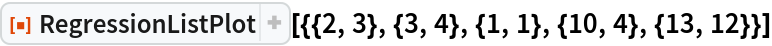Out=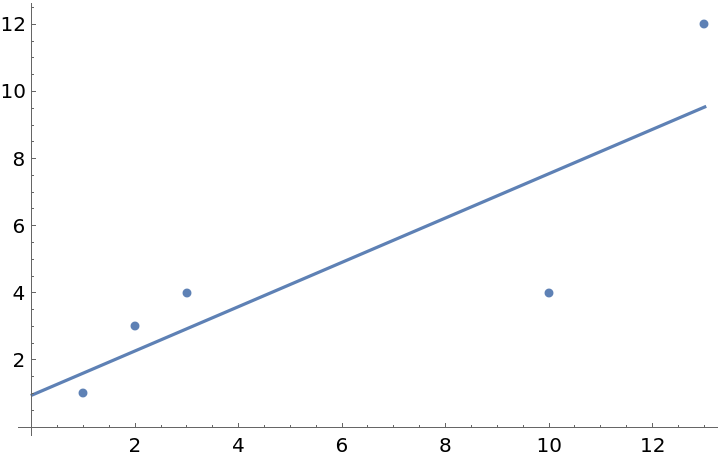### Scope (2)

Generate one dataset of random numbers and one dataset of numbers correlated by a BinormalDistribution:

 In:=Plot both of the examples datasets on the same plot:

 In:=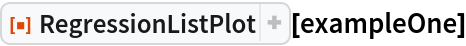Out=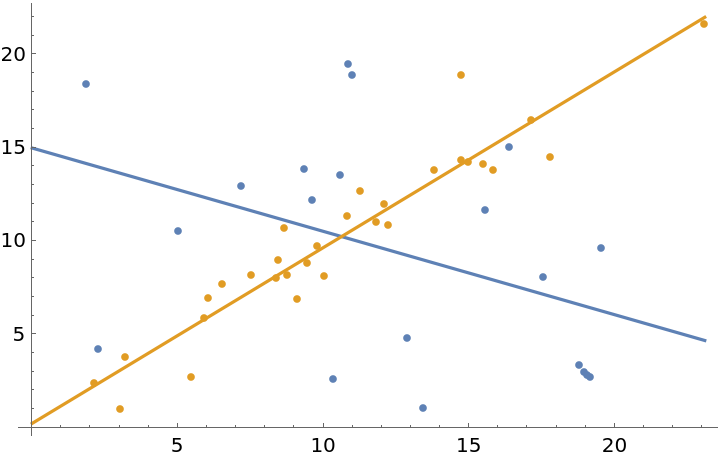### Options (5)

#### Degree (2)

Specify the degree of the regression function using this option:

 In:=In:=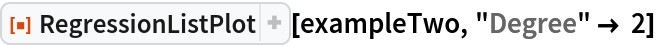Out=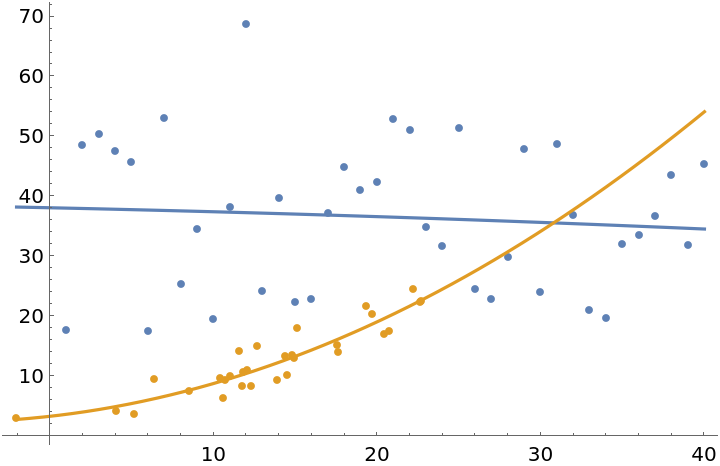Degrees of multiple functions can be specified individually:

 In:=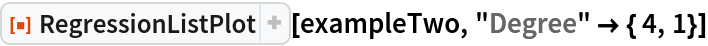Out=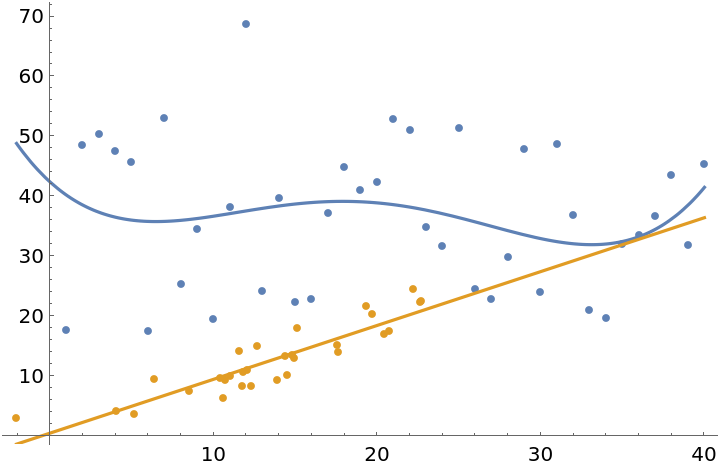#### DisplayEquation (1)

"DisplayEquation"True will display the equation of the regression line:

 In:=Out=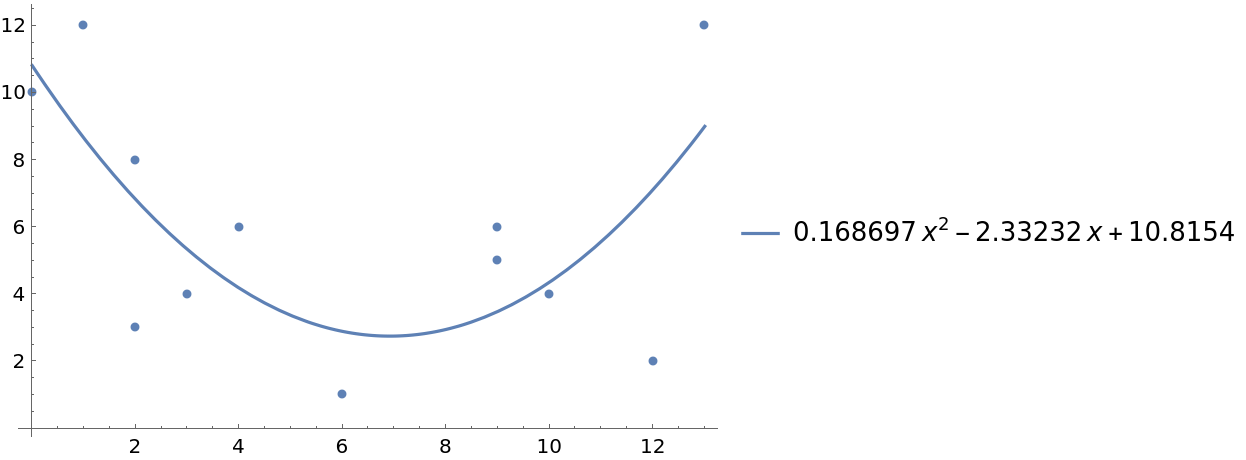#### DisplayRSquared (2)

"DisplayRSquared" specifies whether to display the coefficient of determination. As expected, a random dataset has a much lower coefficient of determination than a related one:

 In:=In:=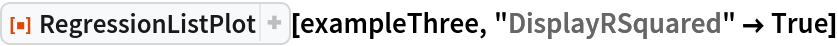Out=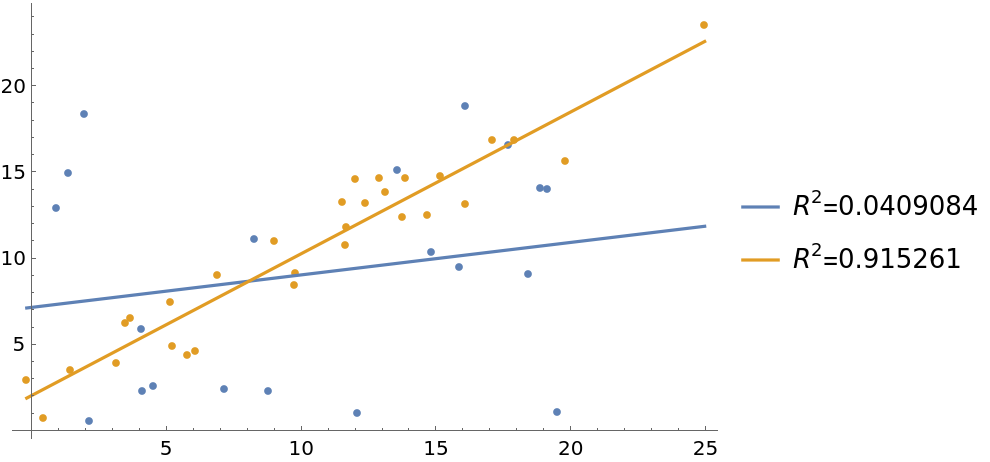"DisplayRSquared" and "DisplayEquation" can both be set to True:

 In:=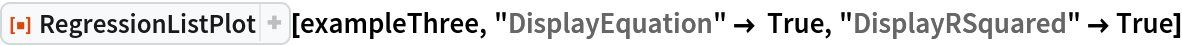Out=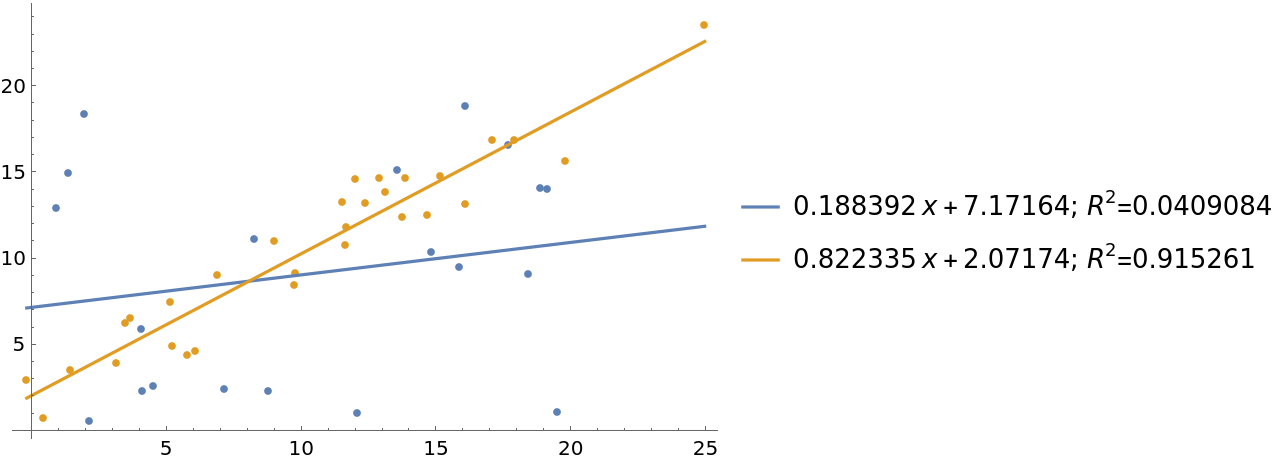### Neat Examples (2)

Correlate the current eruption duration and time to next eruption of Old Faithful geyser, October 1980:

 In:=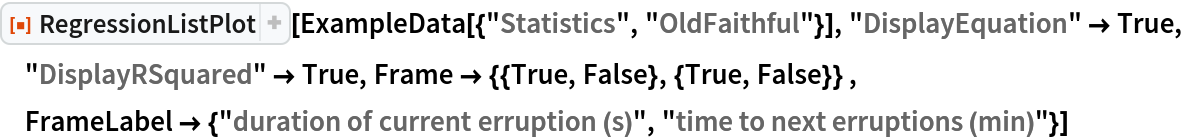Out=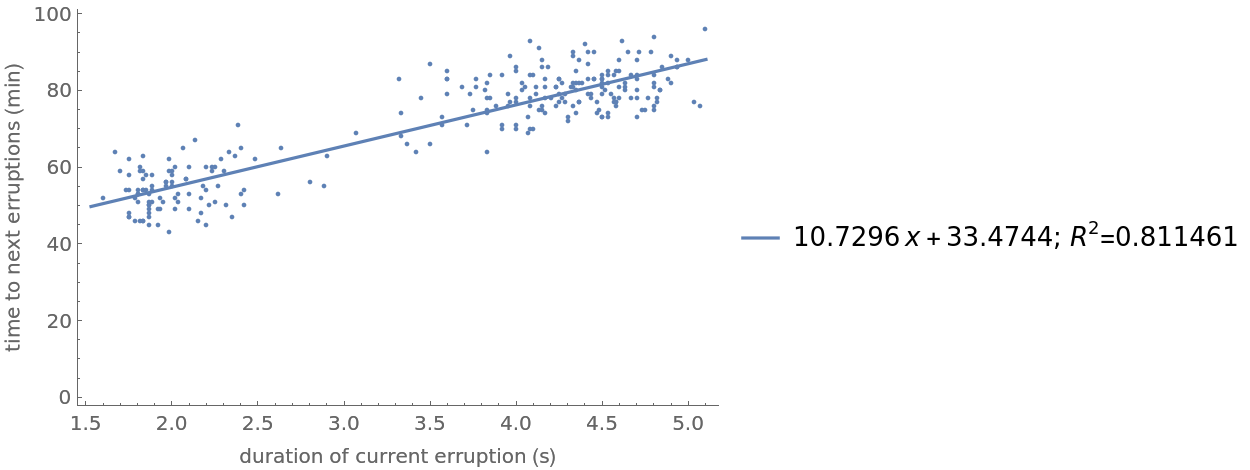Visualize how sepal length, sepal width and petal length are correlated with petal width in the famous Fisher Iris dataset:

 In:=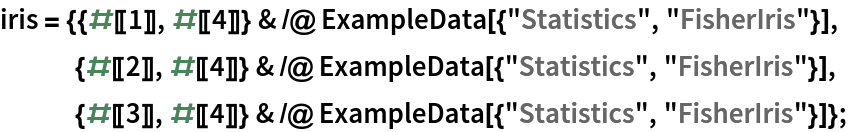In:=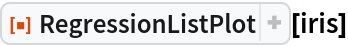Out=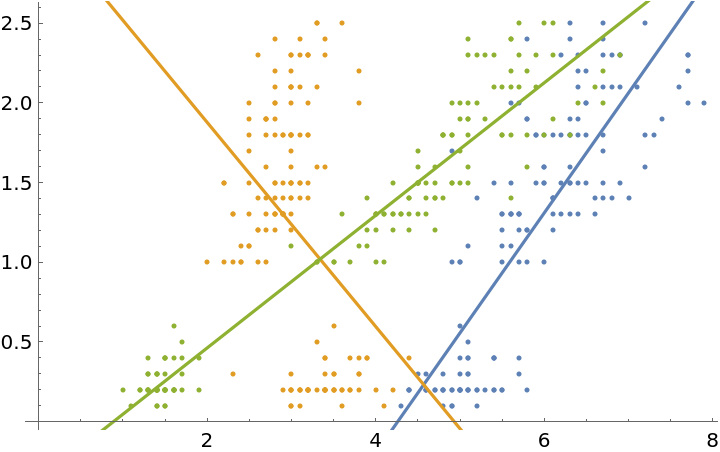## Version History

• 1.0.0 – 24 December 2019